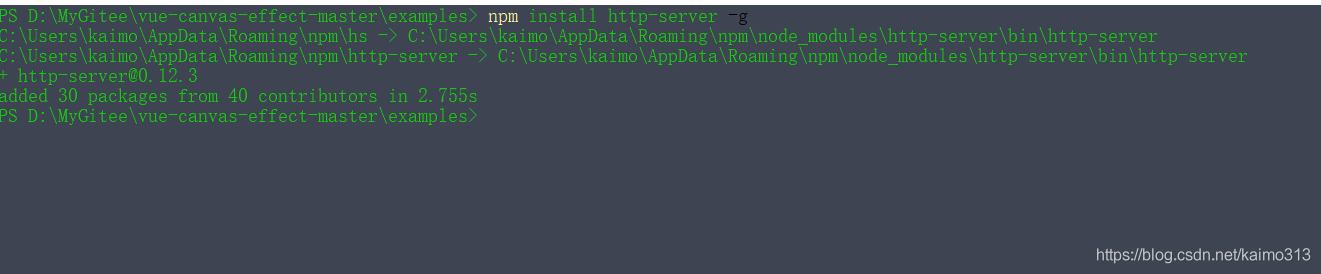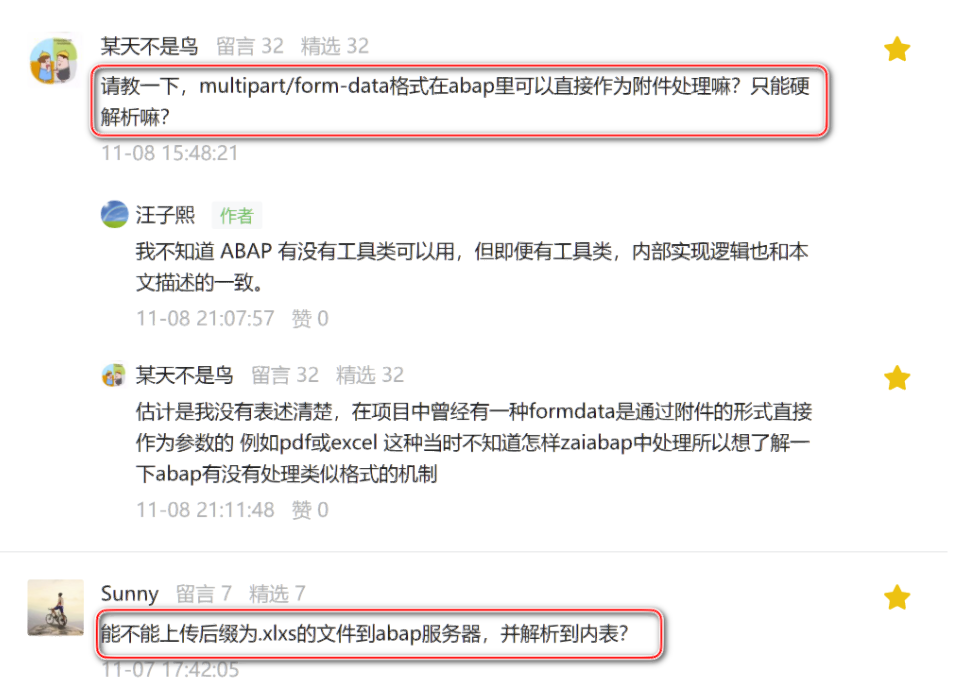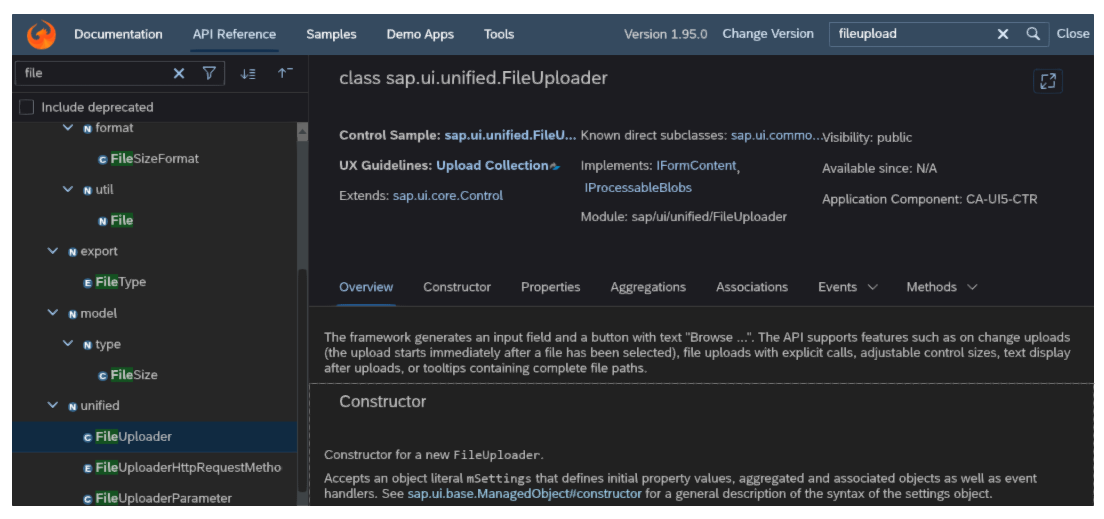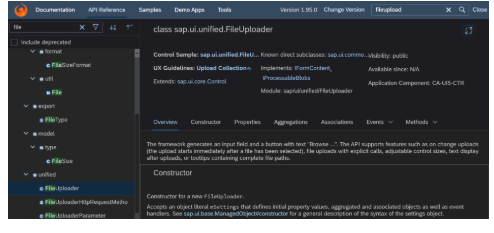# javascript小技巧：同步服务器时间、同步倒计时

+关注继续查看

 1 2 3 4 5 6 7 8 9 10 11 12 13 14 15 16 17 18 19 20 21 22 23 24 25 26 27 28 29 30 31 32 33 34 35 36 37 38 39 40 <span id="timebox">11:21:55 //第一次将服务器时间显示在这里   <script type="text/javascript">     $(function () {  var oTime =$("#timebox");         var ts = oTime.text().split(":", 3);         var tnums = [parseInt(ts), parseInt(ts), parseInt(ts)];         setInterval(function () {             tnums = getNextTimeNumber(tnums, tnums, tnums);             showNewTime(tnums, tnums, tnums);         }, 1000);           function showNewTime(h, m, s) {             var timeStr = ("0" + h.toString()).substr(-2) + ":"                             + ("0" + m.toString()).substr(-2) + ":"                             + ("0" + s.toString()).substr(-2);             oTime.text(timeStr);         }           function getNextTimeNumber(h, m, s) {             if (++s == 60) {                 s = 0;             }               if (s == 0) {                 if (++m == 60) {                     m = 0;                 }             }               if (m == 0) {                 if (++h == 24) {                     h = 0;                 }             }               return [h, m, s];         }     }); 
代码很简单在此就不多作说明(我上面只显示时分秒，大家也可以加上日期，加上日期可在当h==0时，直接从服务器获取一个日期或完整的时间，作为一次时间的校对)，不懂的可以在下面评论，我会及时回复的，然后按照这种思路来实现一下同步倒计时，首先说明一下，什么是同步倒计时，就是类似秒杀一样，设置一个结束时间，然后计算当前时间与结束时间之间间隔，而且必需保证在不同的电脑、浏览器上显示的倒计时时间均相同，实现代码如下：
 1 2 3 4 5 6 7 8 9 10 11 12 13 14 15 16 17 18 19 20 21 22 23 24 25 26 27 28 29 30 31 32 33 34 35 36 37 38 39 40 41 42 43 44 45 46 47 48 49 50 51 52 53 54 55 56 57 58 59 60 61 62  <html> <head>     <title>同步倒计时     <script type="text/javascript" src="jquery-1.4.4.min.js">  <body>     <span id="timebox">1天00时00分12秒      <script type="text/javascript">         $(function () {  var tid = setInterval(function () {  var oTimebox =$("#timebox");                 var syTime = oTimebox.text();                 var totalSec = getTotalSecond(syTime) - 1;                 if (totalSec >= 0) {                     oTimebox.text(getNewSyTime(totalSec));                 } else {                     clearInterval(tid);                 }               }, 1000);               //根据剩余时间字符串计算出总秒数             function getTotalSecond(timestr) {                 var reg = /\d+/g;                 var timenums = new Array();                 while ((r = reg.exec(timestr)) != null) {                     timenums.push(parseInt(r));                 }                 var second = 0, i = 0;                 if (timenums.length == 4) {                     second += timenums * 24 * 3600;                     i = 1;                 }                 second += timenums[i] * 3600 + timenums[++i] * 60 + timenums[++i];                 return second;             }               //根据剩余秒数生成时间格式             function getNewSyTime(sec) {                 var s = sec % 60;                 sec = (sec - s) / 60; //min                 var m = sec % 60;                 sec = (sec - m) / 60; //hour                 var h = sec % 24;                 var d = (sec - h) / 24;//day                 var syTimeStr = "";                 if (d > 0) {                     syTimeStr += d.toString() + "天";                 }                   syTimeStr += ("0" + h.toString()).substr(-2) + "时"                             + ("0" + m.toString()).substr(-2) + "分"                             + ("0" + s.toString()).substr(-2) + "秒";                   return syTimeStr;             }           });http-server：一个JavaScript服务器
http-server：一个JavaScript服务器
8 0JS获取服务器日期，自动切换网页信息
JS获取服务器日期，自动切换网页信息
46 0Node.js 创始人：Javascript 容器是简化服务器抽象的“先驱”践行者
Node.js 创始人：Javascript 容器是简化服务器抽象的“先驱”践行者
44 0Node.js 创始人：Javascript 容器是简化服务器抽象的“先驱”践行者
Node.js 创始人：Javascript 容器是简化服务器抽象的“先驱”践行者
67 0JavaScript 服务器推送技术之 WebSocket

91 0191 070 0121 0137120326

JavaScript 自学手册文档教程322765

Linux Web服务器Nginx搭建与配置136565

JavaScript入门与实战19206529881

Javascript异步编程

Javascript中的对象

JS零基础入门教程（上册）